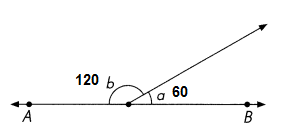This handy Math in Focus Grade 5 Workbook Answer Key Chapter 12 Angles provides detailed solutions for the textbook questions.

Math Journal

Question 1.
$$\overleftrightarrow{X Y}$$ is a line.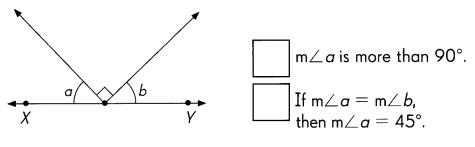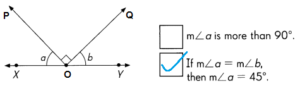Explanation:
The XY is a line and the angle on a straight line is 180°, known as straight angle.
Angle POQ is 90° as per the given information in the diagram.
180° – 90° = 90°
Angle ∠XOP and ∠YOQ are equal angle = 45°

Question 2.
$$\over left right arrow{A B}$$ and $$\over left right arrow{C D}$$ meet at O.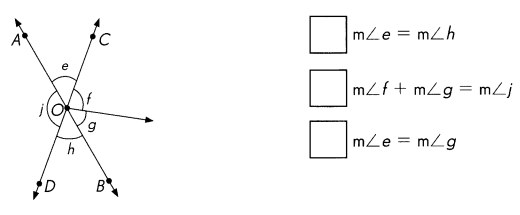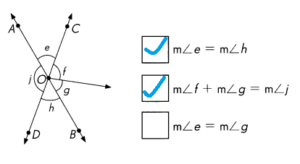Explanation:
$$\over left right arrow{A B}$$ is a line and $$\over left right arrow{C D}$$ is a line are crossed at O, the opposite angles are same.
So, m∠e = m∠h and
m∠f + m∠g = m∠j   are true statements.

Challenging Practice

Find the unknown angle measures. Explain.

Question 1.
$$\over left right arrow{G J}$$ is a line. ∠LHK is a right angle. Find the measure of ∠LHJ.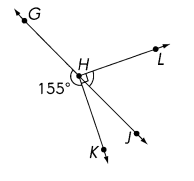Explanation:
Given information
GJ is a straight line, ∠LHK = 90°
∠JHK = 180° – ∠JHK
= 180° – 155° = 25°
∠JHK = ∠LHK – ∠JHK
= 90° – 25° = 65°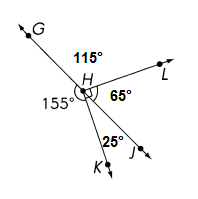Question 2.
$$\over left right arrow{M N}$$ and $$\over left right arrow{X Y}$$ meet at O and m ∠a = m ∠b. Find the measure of ∠c.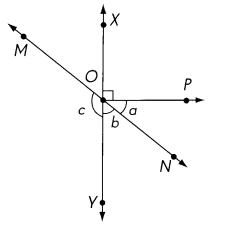Explanation:
$$\over left right arrow{X Y}$$ is a line and $$\over left right arrow{M N}$$ is a line are crossed at O, the opposite angles are same.
given information m∠a = m∠b and ∠XOP = 90
as XOY is a straight line, the angle is 180°
90° + m∠a + m∠b = 180°
∠c = 180° -∠XOM= 180 – 45° = 135°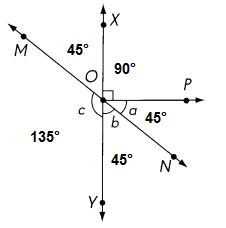Question 3.
$$\over left right arrow{A C}$$ is a line. ∠ABE and ∠DBF are right angles. Find the measure of ∠FBC.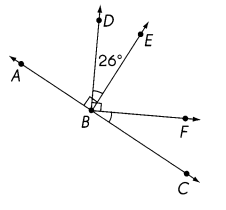Explanation:
∠ABE and ∠DBF = 90°
∠EBF = ∠DBF – ∠DBE
=  90° – 26° = 64°
∠ABD = ∠ABE – ∠DBE
= 90° – 26° = 64°
∠FBC = 180 – (∠ABE + ∠EBF)
= 180° – (90° + 64°)
=180° – 154° = 26°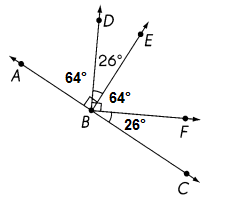Question 4.
$$\over left right arrow{A B}$$ and $$\over left right arrow{W X}$$ meet at O. ∠YOX are right angles. Find the measures of ∠AOX and ∠COY.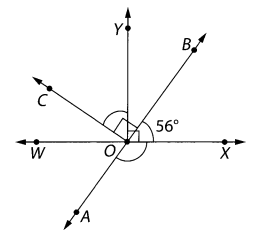∠AOX  = 124°
∠COY  =  56°
Explanation:
90° – 56° = 34°
90° – 34° = 56°
∠COY = ∠COB – ∠BOY
= 90° – 34° = 56°
∠AOX = ∠WOX – ∠AOW
=180°- 56° = 124°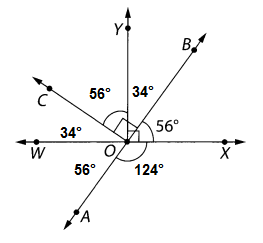Problem Solving

Solve.

Question 1.
$$\over left right arrow{J K}$$ and $$\over left right arrow{L M}$$ are lines.
Check the box for each correct statement.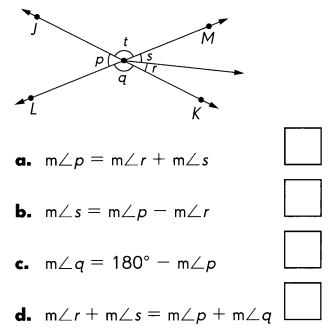$$\over left right arrow{J K}$$ and $$\over left right arrow{L M}$$ are lines.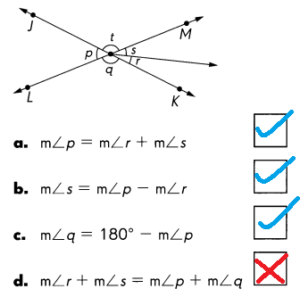Explanation:
$$\over left right arrow{J K}$$ is a line and $$\over left right arrow{L M}$$ is a line are crossed at O, the opposite angles are same.
so, m∠r + m∠s = m∠p + m∠q is the wrong statement.

Question 2.
$$\over left right arrow{A B}$$, $$\over left right arrow{C D}$$, and $$\over left right arrow{E F}$$ meet at O. Find the sum of the measures of ∠AOC, ∠FOD, and ∠BOE.
m∠AOC + m∠FOD + m∠BOE = _____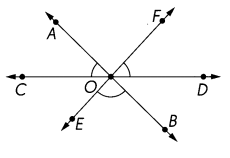Explanation:
m∠AOC = 45°

m∠FOD = 45°

m∠BOE = 90°

m∠AOC + m∠FOD + m∠BOE = 180°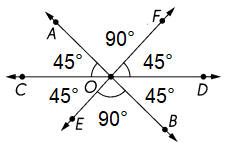Question 3.
ABCD is a square. $$\over right arrow{B E}$$ is a ray. Find the measure of ∠x.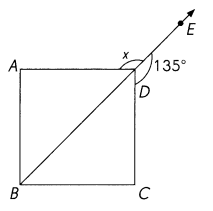Explanation:
As BE is a straight line and the angle is 180° at point D
and a square is ABCD is with 90° angle formed at point D
the ange ∠EDC is 135° angle ∠CDB is 45° and ∠ADB is also 45°
now the
=  180° – 45°  =  135°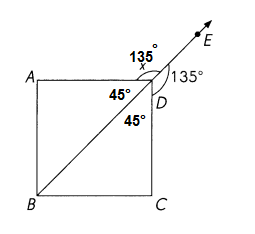Question 4.
How many degrees does the hour hand of a clock turn between 3 P.M. and 7:30 P.M.?
Explanation: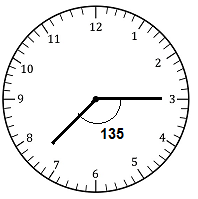Hour hand at 3PM is at 3 and 7:30 as shown in the clock diagram
Total angle is 360°, keep it in mind
and 360° divide in 12 parts
each part is of 30°
from hours hand 3 to 7 :30 its 135°

Question 5.
$$\over left right arrow{A B}$$ is a line. The measures of ∠a and ∠b are whole numbers.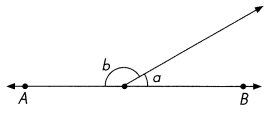If the measure of ∠b is twice that of ∠a, find the measures of ∠a and ∠b.